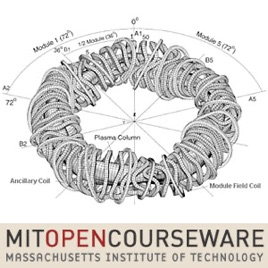22 episodes

This video course covers the fundamental concepts and computer implementations of finite element analysis for nonlinear systems, with examples taken from nuclear, civil, mechanical, aeronautical, and other fields of engineering.# Finite Element Procedures for Solids and Structures, Nonlinear Analysis MIT

• Technology

This video course covers the fundamental concepts and computer implementations of finite element analysis for nonlinear systems, with examples taken from nuclear, civil, mechanical, aeronautical, and other fields of engineering.

• video
Lecture 1: Introduction to nonlinear analysis

## Lecture 1: Introduction to nonlinear analysis

This introductory video discusses nonlinear finite element models in engineering. 4 classes of problem are defined, with examples, and 3 formulations for solution. The basic incremental solution procedure is applied to static and dynamic problems.

• 44 min
• video
Lecture 2: Basic considerations in nonlinear analysis

## Lecture 2: Basic considerations in nonlinear analysis

This lecture introduces the principle of virtual work in nonlinear problems, relating it to the governing equations and boundary conditions of the system. Two examples are analyzed: a plate with a hole, and a plate with a crack.

• 1 hr 5 min
• video
Lecture 3: Lagrangian continuum mechanics variables for analysis

## Lecture 3: Lagrangian continuum mechanics variables for analysis

Nonlinear systems under arbitrary large stretching and rotation can be analyzed using a Lagrangian expression. This lecture introduces the stress, strain, deformation gradient, and deformation tensors commonly used in such analysis, with examples.

• 1 hr 18 min
• video
Lecture 4: Total Lagrangian formulation - incremental analysis

## Lecture 4: Total Lagrangian formulation - incremental analysis

Previously, we derived incremental displacements from the principle of virtual work. The virtual work equation can be linearized with the tensors from lecture 3. The total Lagrangian uses time=0 as a reference, while the updated Lagrangian has time=t.

• 48 min
• video
Lecture 5: Updated Lagrangian formulation - incremental analysis

## Lecture 5: Updated Lagrangian formulation - incremental analysis

The updated Lagrangian uses the same decomposition, linearization, iteration, and discretization procedure as the total Lagrangian (lecture 4), but each derivative is evaluated at time t, not time 0. One method is the modified Newton-Raphson iteration.

• 50 min
• video
Lecture 6: Formulation of finite element matrices

## Lecture 6: Formulation of finite element matrices

Having developed the incremental continuum mechanics equations, this lecture discusses the use of finite element matrices in static, dynamic, and materially-nonlinear-only analysis. Examples of numerical integration techniques are given.

• 44 min

## Customer Reviews

TheLearner ,

### Amazing!!!

If you are interested in Finite Element Analysis these lectures are truly great and worth watching.
They are especially valueable for beginners but, I think, even more advanced persons, in this field, can benefit from watching
these lectures.
Even though they are somewhat dated they still retain their educational value. They are very dense in educational content and very efficient in sharing it with the viewer through good presentations, pictures, and examples.
Not to mention that these lectures are given by a truly amazing Professor who, at the same time, is considered a pioneer and one of the top names in the field.